Latest Banking jobs   »

# Quantitative Aptitude Quiz For LIC ADO Mains 2023- 18th April

Directions(1 – 5): In the given questions, two quantities are given, one as ‘Quantity I’ and another as ‘Quantity II’. You have to determine relationship between two quantities and choose the appropriate option:

Q1. Ratio between length and breadth of rectangle is 7 : 4 and perimeter of rectangle is 88 cm.
Quantity I – Perimeter of square
Side of square is 6 cm less than length of rectangle.
Quantity II – Circumference of the circle
(a) Quantity I > Quantity II
(b) Quantity I < Quantity II
(c) Quantity I ≥ Quantity II
(d) Quantity I ≤ Quantity II
(e) Quantity I = Quantity II or no relation

Q2. Train ‘P’ running at the speed of 72 km/hr cross a man who running in opposite direction at the speed of 9 km/hr in 20 sec.
Quantity I – Length of platform
Length of train ‘Q’ is 70 meter more than that of train ‘P’ and is running at the speed of 90 km/hr and crosses a platform in 40 sec.
Quantity II – 520 m
(a) Quantity I > Quantity II
(b) Quantity I < Quantity II
(c) Quantity I ≥ Quantity II
(d) Quantity I ≤ Quantity II
(e) Quantity I = Quantity II or no relation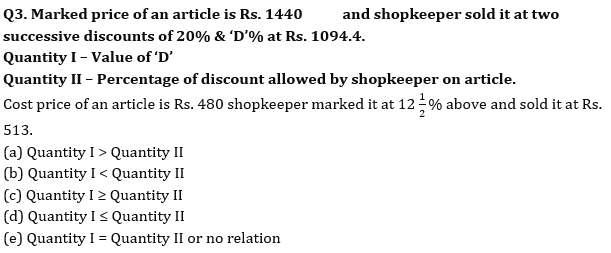Q4. A bag contains seven red balls, three green balls and ‘y’ blue balls. If one ball drawn at random probability of being blue is 3/8.
Quantity I – Probability of all three balls are of different colour.
Three balls taken out from bag at random,
Quantity II – 11/40
(a) Quantity I > Quantity II
(b) Quantity I < Quantity II
(c) Quantity I ≥ Quantity II
(d) Quantity I ≤ Quantity II
(e) Quantity I = Quantity II or no relation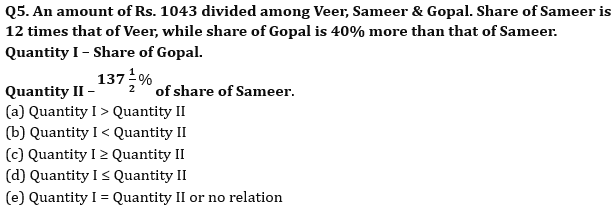Directions (6-10): In the given questions, two quantities are given one as ‘Quantity I’ and another as ‘Quantity II’. You have to determine relationship between two quantities and choose the appropriate option.
Q6.Quantity I: A train can cross a pole in 24 sec with a speed of 75 km/h. Length of train.
Quantity II: A train can cross a pole in 12 sec and a tunnel in 55.2 sec. If length of tunnel is 1800 m. length of train.
(a) Quantity I > Quantity II
(b) Quantity I < Quantity II
(c) Quantity I ≥ Quantity II
(d) Quantity I = Quantity II or no relation
(e) Quantity I≤ Quantity II
Q7. Quantity I: Marked price of article, if article is marked at 50% above cost price and on selling the article, profit earned is 20% and S. P is Rs 1020.
Quantity II: Total cost of fencing a square of side 37.5 meter and cost of wire is Rs 0.17 per centimeter.
(a) Quantity I > Quantity II
(b) Quantity I < Quantity II
(c) Quantity I ≥ Quantity II
(d) Quantity I = Quantity II or no relation
(e) Quantity I≤ Quantity II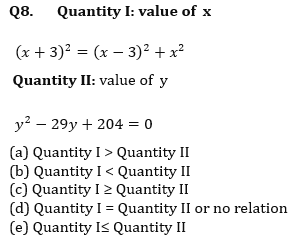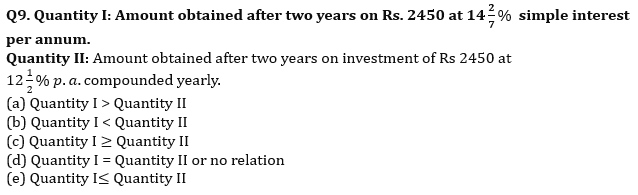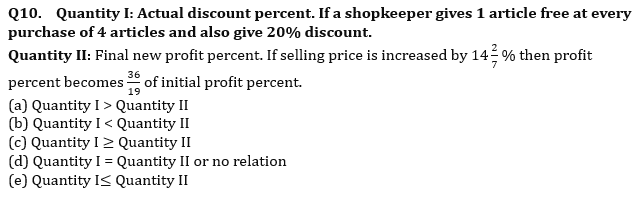Directions (11 -15): Given below in each question there are two statements (I) and (II). You must determine; which statement is enough to give the answer of question. Also, there are five alternatives given, you have to choose one alternative as your answer of the questions:

Q11. What is area of the rectangle.
I. Length is 50% more than breadth.
II. Perimeter of square is 48 cm and breadth of rectangle is equal to side of square.
(a) only statement I
(b) Only statement II
(c) Both I and II together
(d) Either I or II alone
(e) Both statements together are not sufficient

Q12. What is age of Rahul after 2 years.
I. Average age of Arun and Neeraj is 24 years and ratio of age of Rahul to Arun is 2 : 3.
II. Neeraj is 4 years elder than Satish and ratio of age of Satish to Rahul is 1 : 2
(a) only statement I
(b) Only statement II
(c) Both I and II together
(d) Both statements together are not sufficient
(e) Either I or II alone

Q13. What is the speed of boat in still water when the upstream speed of boat is equal to the speed of stream?
I. Time required to cover certain distance upstream is 24 sec.
II. Time required to cover certain distance downstream is 8 sec.
(a) only statement I
(b) Only statement II
(c) Both I and II together
(d) Both together are not sufficient
(e) Either I or II alone

Q14. Find out the length of train X given that speed of train X is 20 m/sec.
I. Train X crosses another train Y moving in opposite direction in 6 sec and the speed of train Y is 50% more than the speed of train X.
II. Length of train Y is 50% less than length of train X.
(a) Both I and II together
(b) Only statement I
(c) Only statement II
(d) Both I and II together are not sufficient
(e) Either I or II alone

Q15. What is the total strength of company Adda247.
I. Ratio of male to female employees are 1 : 2
II. Total females are 280 and males are 50% of female.
(a) Both I and II together
(b) Only statement I
(c) Only statement II
(d) Both I and II together are not sufficient
(e) Either I or II alone

Solutions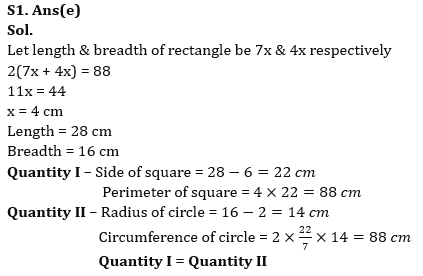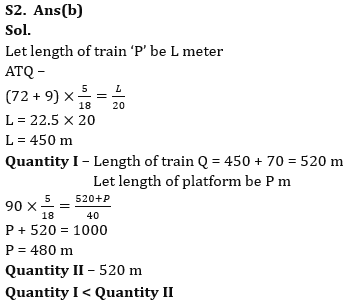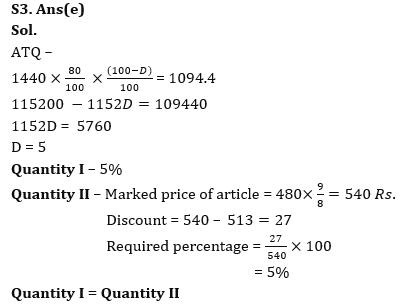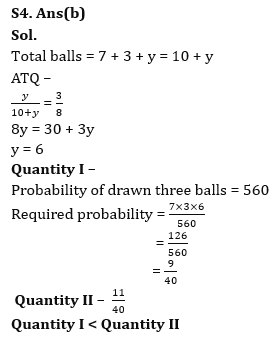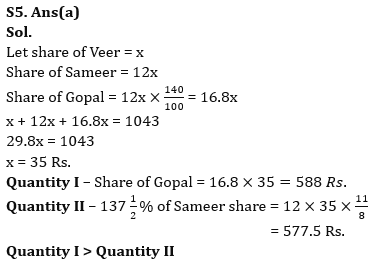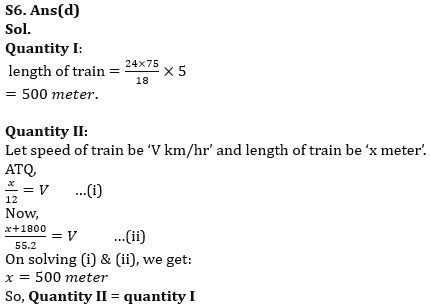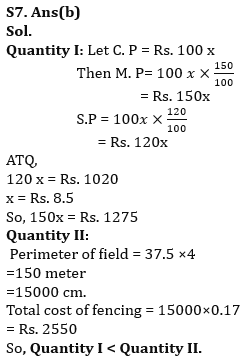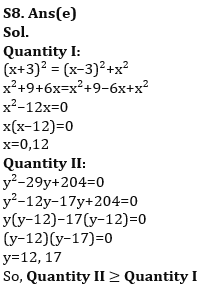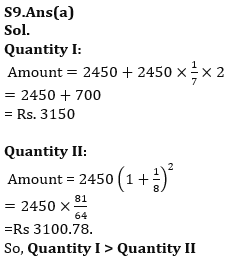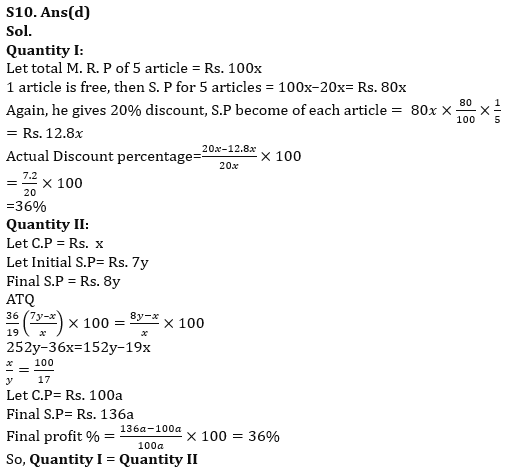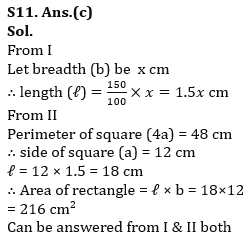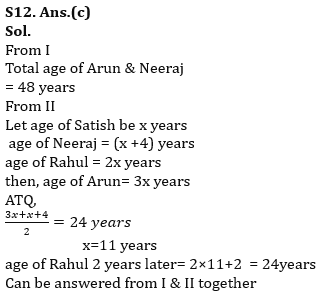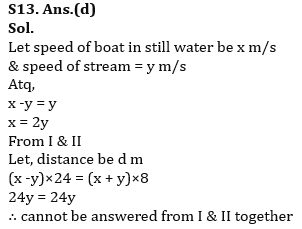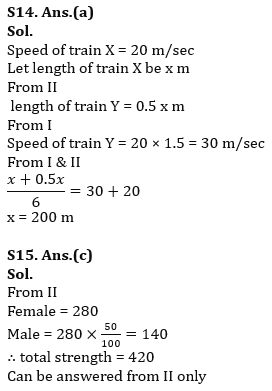## FAQs

### When is the LIC ADO Mains Exam scheduled?

The LIC ADO Mains exam is scheduled to be held on 23 April 2023

#### Congratulations!Union Budget 2023-24: Free PDF